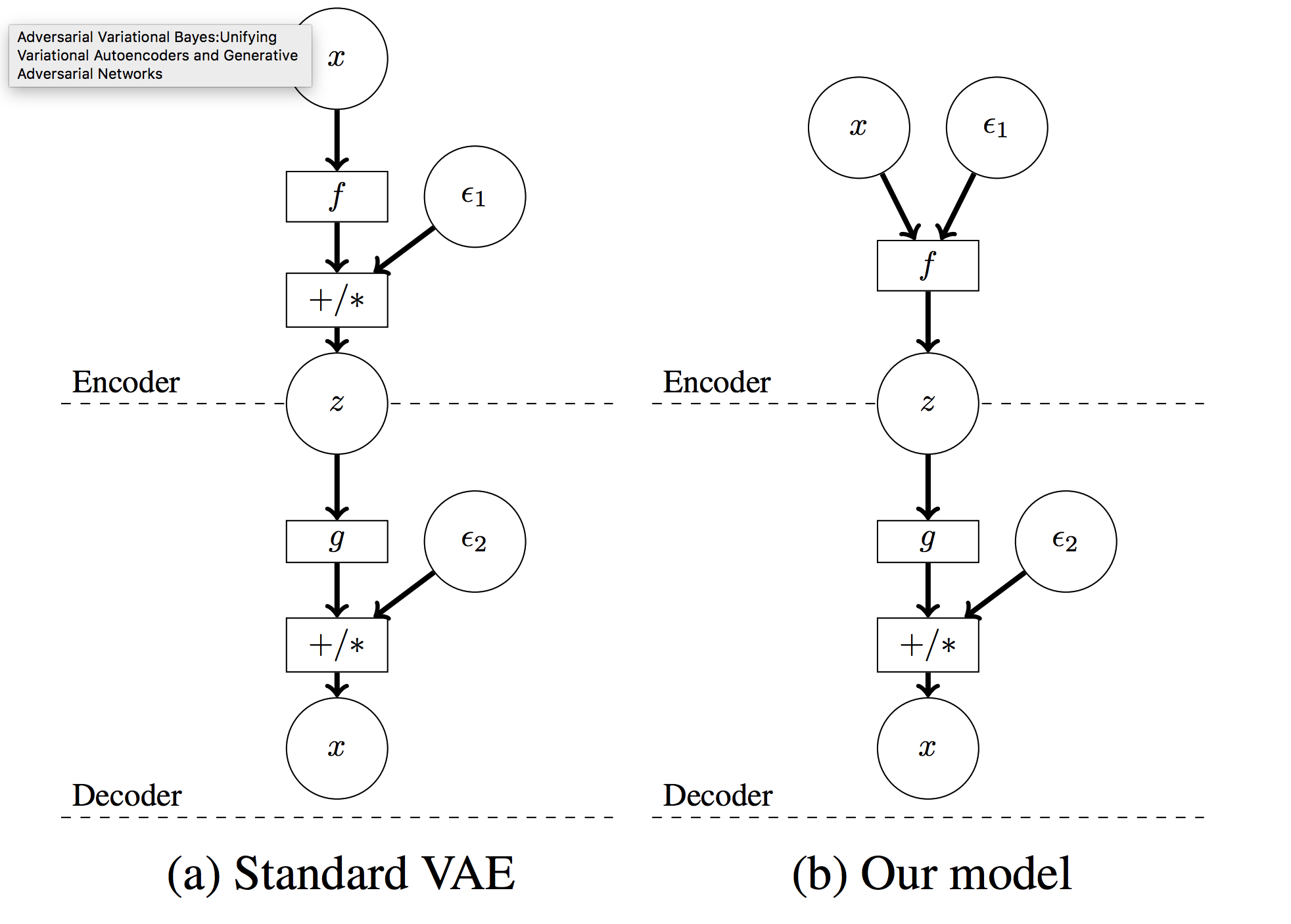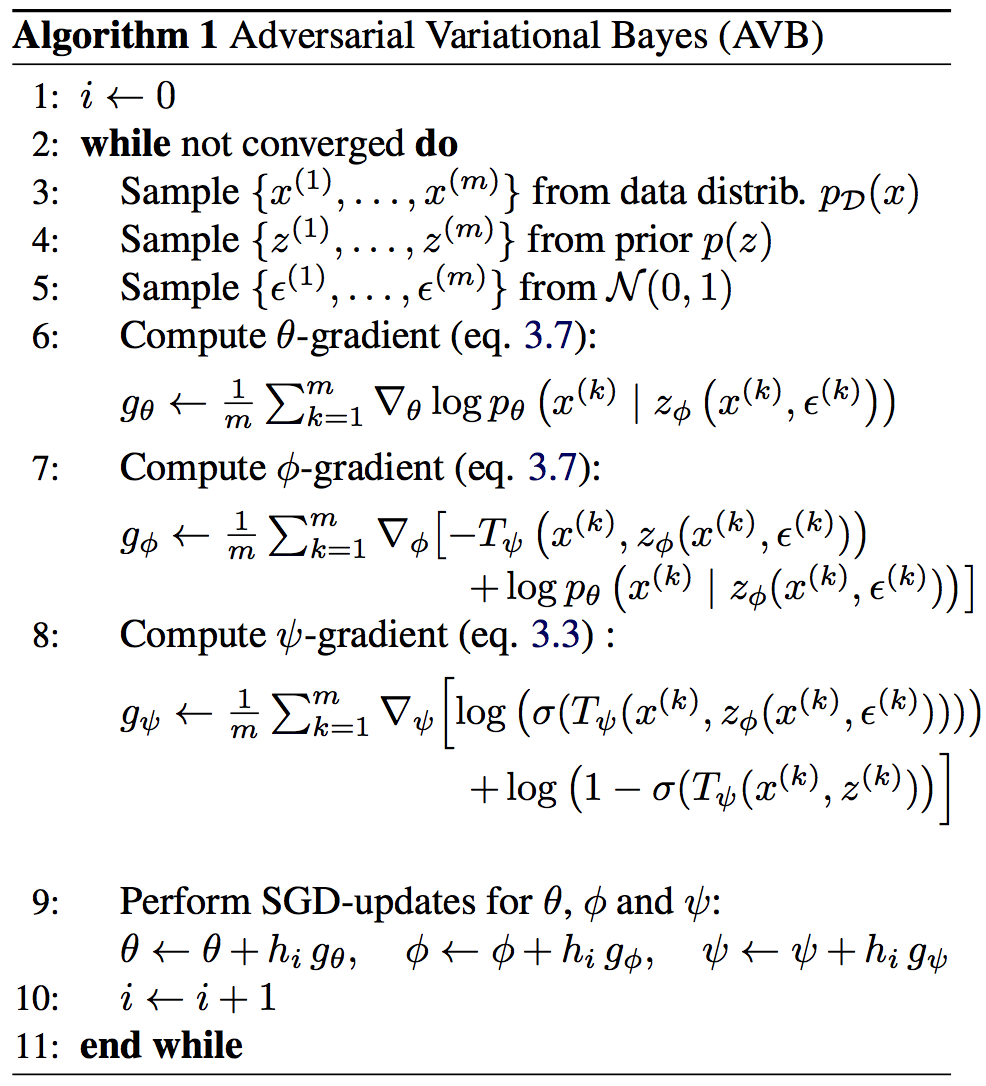## WHY?

In VAE framework, the quality of the generation relies on the expressiveness of inference model. Restricting hidden variables to Gaussian distribution with KL divergence limits the expressiveness of the model.

## WHAT?Adversarial Variational Bayes apply gan loss to VAE framework. AVB is different from former VAE in 2 ways: x is inserted to the encoder with noise(epsilon) and the encoder output z. Also, instead of KL divergence, discriminator is used to keep z to the prior. The second difference seems similar to that of AAE, but key difference is that the discriminator distinguishes pairs of x and z from pairs of x and priors. Algorithm is as follows.Discriminator may not work well in the case when two densities are very differnt. This paper use a technique called “Adaptive Contrast” that provide auxiliary conditional probability distribution r and calculate the contrast between r and q. Therefore, variational lowerbound is
$E_{P_{mathcal{D}}}(x)[-KL(q_{\phi})(z|x, r_{\alpha}(z|x)) + E_{q_{\phi}(z|x)}(-logr_{\alpha}(z|x)+logp_{\phi}(x, z))]$
Models are trained to maximize
$E_{P_{mathcal{D}}}E_{q_{\phi}(z|x)}(-T^*(x,z) -logr_{\alpha}(z|x)+logp_{\phi}(x, z))$

## So?

AVB was able to capture more complex posterior distribution and resulted in much better log liklihood and KL divergence in MNIST.

## Critic

Interesting idea. Planned to implement with Pytorch soon.

Mescheder, Lars, Sebastian Nowozin, and Andreas Geiger. “Adversarial variational bayes: Unifying variational autoencoders and generative adversarial networks.” arXiv preprint arXiv:1701.04722 (2017).